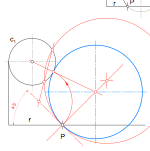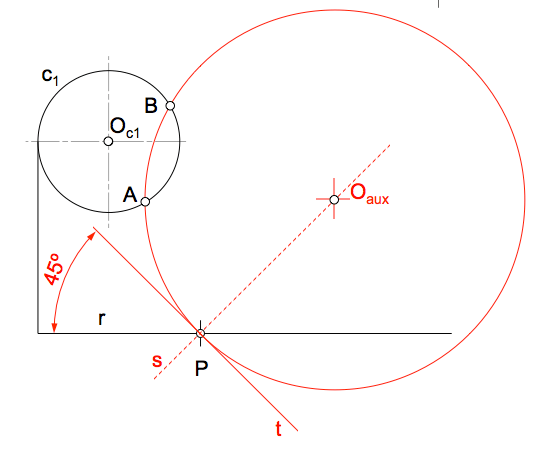# Metric geometry: Circunferencias con condiciones angulares. Solución al Problema IOf the various solutions that can be given to problem proposed obtaining circles with angular conditions ( passing through a point, are tangent to a circle, forming an angle with a straight), we will analyze this solution using the application of Power Concepts used in the “Fundamental Problem Tangencies” ( PFT ).

Later we will discuss specific ways to this particular problem that could simplify or geometric layout conceptualization.

In the latter regard it is noteworthy that a geometric construction given, a set of lines, can be interpreted in different ways in response to abstract reasoning applied to the problem.

The general model search can be the first step of a surveyor training.

## The amendment of the problem statement

The first step, applying geometric logical method or working methodology exposed, consist change the geometric conditions of the problem for others that are equivalent.

Generally, try to impose the same conditions in the case of restrictions to convert angular restrictions “isoangularidad”. In this case, change the condition of an angle of 45 ° with a straight line by being tangent to another, as we have a condition of tangency circumference. We see that the statement will change to:

Determining a circle which is tangent to a line and a circle and pass (es tangente) by a point.

Similarly it could be changed by a tangency condition to 45 ° angle, although this concept now seems more complex than the way employee.

## Statement modified equivalent

Indeed, if the desired circumference forms an angle with the straight r, su tangent t at the point of contact should be the angle r, as we saw in defining the angle between straight and circumference.

Our problem consists therefore in determining a tangent to another and a line on one of its points.

Isogonalidad The problem that remains is one of the variants known as “Problem of Apollonius” proposing the determination of a circle tangent to three given circles.

Three circles? Indeed, crossing point can be considered as a circle of radius zero (null) and the line “t” otra de radio infinity. This type of reasoning can group therefore this problem in a more general simply, as posed at the beginning.

Your solution may therefore be inferred from the general model, with the corresponding generalization, or simplifications can be included due to the nature of the restrictions.

## Particularized approach to solution

The circles tangent to the line t point P will have its center on a line perpendicular to t through point P. Determine a parabolic beam circumferences radical axis with the line t.

The line s es el locus of the centers of circles which are tangent to the line r point P.Finally determine the center of the circle solution (blue) supplementing the problem. To do determine the circumference that is tangent to the line at the point P t is tangent to the circumference turn c1,

If we determine a circle whatever t tangent to the line at the point P, and it intersects the circumference c1 on a couple of points (A and B), we will be getting one of the circumferences of said parabolic beam.

Point “I” of intersection of the line AB and the line t radical is the center of the circles tangent to t and passing through A and B, thereby having equal power respect of all. This power value is the distance to the P squared tangent, and therefore allows determining the point T tangency in c1.Missing number analysis of solutions to the problem of determining generic circles to form an angle with the straight, passing through a point and are tangent to the circumference. Of possible solutions that will always come in pairs, we choose the one that suits the sketch given in the statement.

Overall, a problem of tangents on three circles, (Problem of Apollonius), we will have to 8 solutions. In this case are limited to two in degenerate circumference straight and the other in a point.

Can you solve this exercise with a different model? Best to do many of the same exercises, tanganika solve it in many different ways !!!

The types of investment are especially applicable in these problems, as seen in the “Application to the resolution of problems and angular tangents

Metric geometry